Graphing Motion

Let's say that we wish to travel from point A to point B and back again along a walkway that has installed on it a convenient number line. While we are moving, an associate will note where we are at the end of each second. We can record and display our results in a table; this may help organize the data into a form that might lead to deeper understanding
Sample data and corresponding graph.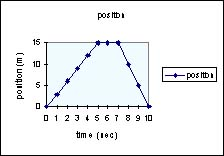pos 0 3 6 9 12 15 15 15 10 5 0 time 0 1 2 3 4 5 6 7 8 9 10

.Our data taker makes the following observations. We are at the starting place at time t = 0. We dash to point B 15 m distant, arriving at our destination at the end of 5 seconds; we then stop to rest (our position does not change) for two more seconds; finally, we race back to arrive at our starting place at the end of the tenth second. A position versus time graph is shown in the box. If that is all there was to graphing, it would not be exciting and would not be included here.
Let's look more closely at what the graph may be hiding. Let's consider the
slope of the curve. Remember that slope is defined as change in vertical coordinate (rise) over the change in horizontal coordinate (run). That means for this graph the slope = rise/ run = change in position divided by the elapsed time. But this is the definition for velocity. In the graph above, for the interval 0-5sec the slope is positive and constant. That means our velocity is positive (pointing to the right by our sign convention). From t = 5 sec to t = 7 sec, the change in position is zero.Therefore, were are not moving. Finally, from t = 7 sec to t = 10 sec our position returns to zero. The slope is negative and larger in absolute value than the slope for the first 5 sec.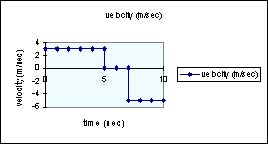A sketch of a velocity vs. time graph looks like the box at left. For simplicity sake we are assuming that the transitions from one speed to another occur in virtually no elapsed time.

THis is a applet that plots position v time, velocity v time, and acceleraion v time

http://www.walter-fendt.de/ph14e/If we graph velocity as a function of time, we get the curve at left. Look at the slope of this graph. It is negative and constant. The slope of a velocity vs. time graph yields acceleration.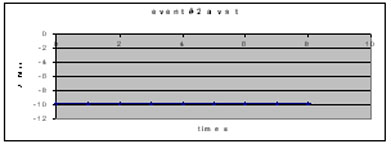At left is a graph of acceleration as a function of time. You will note that the curve is horizontal and stuck on -9.8 m/s^2. This is the expected value for the acceleration of any free-falling object.

Now consider a new event that is closely related to the last event. Let us assume that a perfect super ball ( a ball that loses no energy upon collision with the ground) falls from rest from a high place, strikes the ground and rebounds precisely to its starting place. What would the graphs look like now?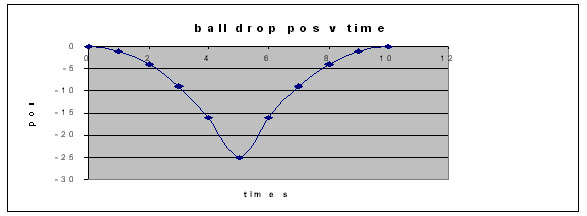At left is the position vs. time graph. notice the very abrupt change in the direction of the curve at about 5 seconds.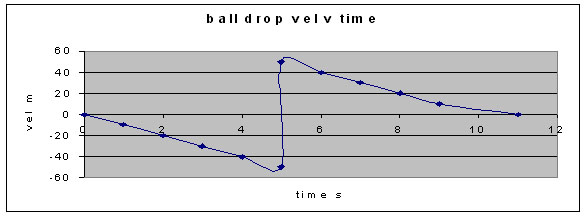Now comes the velocity vs. time graph. Look at what happens to the velocity at 5 seconds. At one moment, the velocity was -50m/s; a moment later, +50 m/sec. The connecting piece is steep but it is not vertical.

Questions

1. For the bouncing ball situation, what would the graphs look like if the ball bounced a second time? Look at the middle parts of these graphs. Ever seen anything like these before?

2. Consider this situation: We toss a ball into the air. Before gravity can stop it, the ball hits the ceiling. Sketch the graphs.